### Home > PC > Chapter 8 > Lesson 8.2.1 > Problem8-72

8-72.
1. Given f(x) =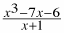. Homework Help ✎

1. What is the value of f (−1)?

2. Find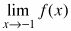. (Hint: polynomial division)

3. Use what you found from part (b) to sketch f(x) without using a calculator.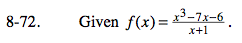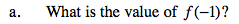What happens when the denominator is 0?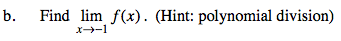Substitute x = −1 into the quotient.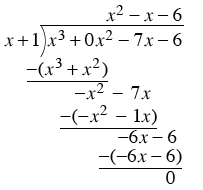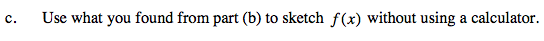You know it is a parabola with a hole at x = −1. Find the x-intercepts by factoring.